# Excel nested if statements with ranges

Many people usually asked that how to write an excel nested if statements based on multiple ranges of cells to return different values in a cell? How to nested if statement using date ranges? How to use nested if statement between different values in excel 2013 or 2016?

This post will guide you how to understand excel nested if statements through some classic examples.

## Nested IF statements based on multiple ranges

Assuming that you want to reflect the below request through nested if statements:

a) If 1<B1<0, Then return 0.1

b) If 0.99<B1<5, then return 0.15

c) If 4.99<B1<15, then return 0.2

d) If 14.99<B1<30, then return 0.5

So if B1 cell was “14.5”, then the formula should be returned “0.2” in the cell.

From above logic request, we can get that it need 4 if statements in the excel formula, and there are multiple ranges so that we can combine with logical function AND in the nested if statements.  The below is the nested if statements that I have tested.

`=IF(AND(B1>0,B1<1),0.1,IF(AND(B1>0.99, B1<5),0.15, IF(AND(B1>4.99,B1<15),0.2, IF(AND(B1>14.99,B1<30),0.5,””))))`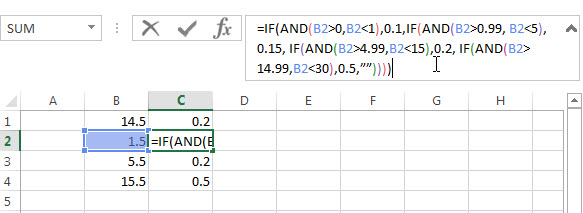If you don’t want to use AND function in the above nested if statement, can try the below formula:

`=IF(B1<1,0.1, If(B1<5,0.15,IF(B1<15,0.2,IF(B1<30,0.5,””))))`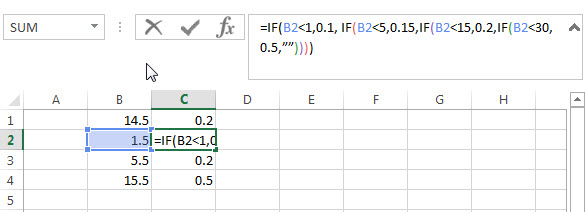## Nested IF Statement Using Date Ranges

I want to write a nested IF statement to calculated the right Quarter based one the criteria in the below table.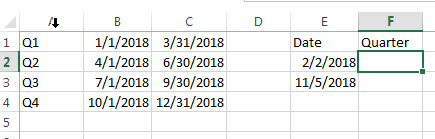According to the request, we need to compare date ranges, such as: if B1<E2<C1, then return A1.

So we can consider to use AND logical function in the nested if statement. The formula is as follows:

`=IF(AND(E2>B1,E2<C1),A1,IF(AND(E2>B2,E2<C2),A2,IF(AND(E2>B3,E2<C3),A3,IF(AND(E2>B4,E2<C3),A4))))`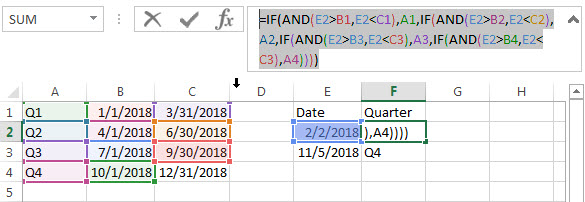Or we can use INDEX function and combine with MATCH Function to get the right quarter.

`=INDEX(\$A\$1:\$A\$4,MATCH(E2,\$B\$1:\$B\$4,1))`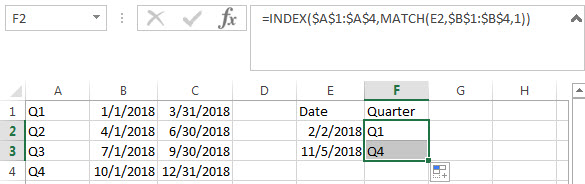## Nested IF Statements For A Range Of Cells

If you have the following requirement and need to write a nested IF statement in excel:

If any of the cells A1 to C1 contain “excelhow”, then return “excelhow” in the cell E1.

If any of the cells A1 to C1 contain “google”, then return “excelhow” in the cell E1.

If any of the cells A1 to C1 contain “ibm”, then return “ibm” in the cell E1.

If any of the cells A1 to C1 contain “Cloud”, then return “ibm” in the cell E1.

How to check if cell ranges A1:C1 contain another string, using “COUNTIF” function is a good choice.

Countif function: Counts the number of cells within a range that meet the given criteria

Let’s try to test the below nested if statement:

`=IF(OR(COUNTIF(A3:C3,"excelhow")>0,COUNTIF(A3:C3,"google")>0),"excelhow",IF(OR(COUNTIF(A3:C3,"ibm")>0,COUNTIF(A3:D3,"cloud")>0),"ibm",""))`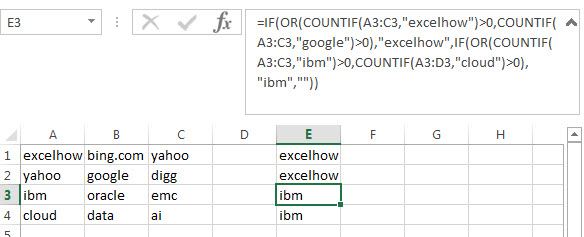## Nested IF Statement between different values

Assuming you have the following different range values, if the B1 has the value 65, then expected to return “under average”in cell C1, if the Cell B1 has the value 75, then return “average” in cell C1. And if the Cell B1 has the value 85, then return “above average” in the cell C1.

 0-70 under average 71-80 average 81-100 above average

How do I format the nested if statement in Cell C1 to display the right value? Just try to use the below nested if function or using INDEX function.

`=IF(B1< 0,"",IF(B1<= 70, "under average",IF(B1<=80, "Average", IF(B1<=100,"Above Average",""))))`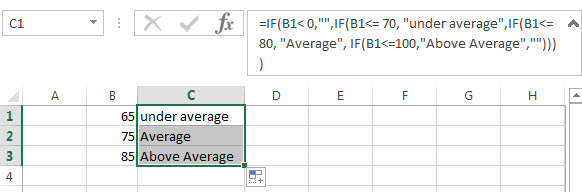### Related Functions

• Excel IF function
The Excel IF function perform a logical test to return one value if the condition is TRUE and return another value if the condition is FALSE. The IF function is a build-in function in Microsoft Excel and it is categorized as a Logical Function.The syntax of the IF function is as below:= IF (condition, [true_value], [false_value])….
• Excel nested if function
The nested IF function is formed by multiple if statements within one Excel if function. This excel nested if statement makes it possible for a single formula to take multiple actions…
• Excel AND function
The Excel AND function returns TRUE if all of arguments are TRUE, and it returns FALSE if any of arguments are FALSE.The syntax of the AND function is as below:= AND (condition1,[condition2],…) …
• Excel COUNTIF function
The Excel COUNTIF function will count the number of cells in a range that meet a given criteria.This function can be used to count the different kinds of cells with number, date, text values, blank, non-blanks, or containing specific characters.etc.The syntax of the COUNTIF function is as below:= COUNTIF (range, criteria) …
• Excel INDEX function
The Excel INDEX function returns a value from a table based on the index (row number and column number)The INDEX function is a build-in function in Microsoft Excel and it is categorized as a Lookup and Reference Function.The syntax of the INDEX function is as below:= INDEX (array, row_num,[column_num])…
• Excel MATCH  function
The Excel MATCH function search a value in an array and returns the position of that item.The MATCH function is a build-in function in Microsoft Excel and it is categorized as a Lookup and Reference Function.The syntax of the MATCH function is as below:= MATCH  (lookup_value, lookup_array, [match_type])….

Related Posts

Find the Closest Data to the Data Provided in Excel

In our daily work, we may encounter such an issue that to find the closest value to a certain value. In fact, Excel internal functions can help us solve this problem. In today’s article, we will show you how to ...

Abbreviate Names Or Words in Excel

As an MS Excel user, you might have come across a task where you need to abbreviate different names or words, and there are also possibilities that you might have done this task manually by assuming that there isn't any ...

Convert State Names To Abbreviations

Assume that you got a task to convert the full state's name into the abbreviations in MS Excel, and for doing this task, you might choose to do it manually, which is an acceptable way only if you don't have ...

Filter or Remove Columns

Assume that in MS Excel, you have a table consisting of a few columns consisting of few values, and you want to filter to remove the specified columns from the table. You might take it easy and would prefer to ...

Filter or Extract for Multiple OR Criteria

If you are an valid MS Excel user, you have probably come across a situation where you wanted to filter the data in a separate table with specific criteria. You could do this task manually, which is also acceptable when ...

Sidebar